# Principal curvature

(diff) ← Older revision | Latest revision (diff) | Newer revision → (diff)

The normal curvature of a surface in a principal direction, i.e. in a direction in which it assumes an extremal value. The principal curvatures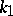and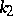are the roots of the quadratic equation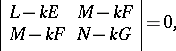(*)

where,andare the coefficients of the first fundamental form, while,andare the coefficients of the second fundamental form of the surface, computed at the given point.

The half-sum of the principal curvaturesandof the surface gives the mean curvature, while their product is equal to the Gaussian curvature of the surface. Equation (*) may be written aswhereis the mean, andis the Gaussian curvature of the surface at the given point.

The principal curvaturesandare connected with the normal curvature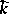, taken in an arbitrary direction, by means of Euler's formula: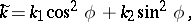whereis the angle formed by the selected direction with the principal direction for.

In the case of an-dimensional submanifoldof Euclidean-space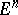principal curvatures and principal directions are defined as follows.
Letbe a unit normal toat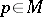. The Weingarten mapping (shape operator)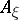ofatin directionis given by the tangential part of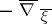, where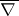is the covariant differential inand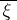is a local extension ofto a unit normal vector field.does not depend on the chosen extension of. The principal curvatures ofatin directionare given by the eigen values of, the principal directions by its eigen directions. The (normalized) elementary symmetric functions of the eigen values ofdefine the higher mean curvatures of, which include as extremal cases the mean curvature as the trace ofand the Lipschitz–Killing curvature as its determinant.